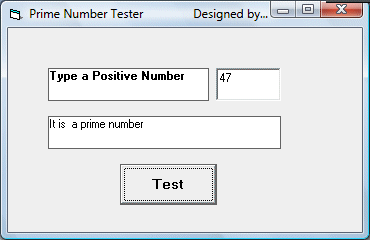# Prime Number Tester

This program can test whether a number entered by the user is a prime number or not. A prime number is a number that cannot be divided by other numbers other than by itself. Examples are 2, 3, 5, 7, 11, 13,17,19, 23,29, 31, 37 and more. In this program, you can use the Select Case  ......End Select statement to determine whether a number entered by a user is a prime number or not. For case 1, all numbers that are less than 2 are prime numbers. In Case 2, if the number is 2, it is a prime number. In the last case, if the number N is more than 2, we need to divide this number by all the numbers from 3,4,5,6,........up to N-1, if it can be divided by any of these numbers, it is not a prime number, otherwise it is a prime number. To control the program flow, we can use the Do......Loop While statement . Besides, we need to tag="Not Prime' to identify the number that is not prime, so that when the routine exits the loop, the label will display the correct answer.

### The Interface#### The Code

Private Sub Command1_Click()
Dim N, D  As  Single
Dim tag As String

N = Val(TxtNumber.Text)

Select Case N
Case Is < 2
Lbl_Answer.Caption = "It is not a prime number"

Case Is = 2
Lbl_Answer.Caption = "It is a prime number"

Case Is > 2
D = 2
Do
If N / D = Int(N / D) Then
Lbl_Answer.Caption = "It is not a prime number"
tag = "Not Prime"
Exit Do
End If
D = D + 1

Loop While D <= N - 1
If tag <> "Not Prime" Then
Lbl_Answer.Caption = "It is a prime number"
End If
End Select
End Sub